• 31
•
•
•
•
•
•
•
31
Shares

# SPRING FORCE

Today we’re going to discuss the springs (not about the season) used in mechanical things, spring force, ideal springs, springs as shock absorbers, and work done by spring force. We all must have seen springs used in toys and many more things. Also if we compress our elongate spring it exerts a force, today we will know the relation about the force exerted by Spring with the amount of elongation/compression, also we will know about the work done by spring force.

## SPRINGS

In our everyday life we came across springs a lot of time, we have experienced that if we compress or elongate a spring it oscillates and finally regain its natural position. The original length of a spring when there is no net force acting on it is natural

## Ideal springs

Since spring’s behavior is different for different springs, we are surrounded by Physics ideal springs for the study.

An ideal spring is a spring that is massless and forces exerted by it is directly proportional to the amount of compression or elongation in spring. Alt the real springs can show deviations from ideal springs but it will be easier to study the ideal springs therefore all the discussion we are making is for ideal springs only.

## Spring Force

If we elongate or compress a spring by let’s start “x” length, we experience a force exerted by spring by experiments. We can observe whether we compress or elongate a spring from natural length by the same amount the force exerted by spring is the same. And higher we elongate or compress higher will be the force.

Also if we elongate spring, we feel that its hand is pulled back by spring i.e the force exerted by spring is opposite to the Direction of elongation or compression.

Summarising above we know that spring’s force is directly proportional to the amount of elongation or compression from the natural length and is in an opposing direction to that of compression or elongation.

Mathematically,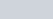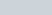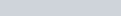Since force should be in a direction opposite to the x vector, k should be positive.

K is proportionality constant and is known as spring constant.

## Spring constant and Springs as shock absorbers

Now, another thing which we observe is that if we compress or elongate different springs by the same amount we will feel that the forces exerted by different springs will be different, which will depend on the nature of spring since the force exerted is still proportional to “x”, therefore from above is clear that spring constant is different for different springs and its characteristic of nature of spring.

We see the uses of springs in our daily life as shock absorbers as used in vehicles or other things . Spring are shock absorbers if one end of it is given a force then it doesn’t transfer the force(change in momentum) suddenly to other end because spring first stores that energy into potential energy of spring and then it releases it and this doesn’t happened suddenly.

## Work done by spring force

We had already studied how work done by a force is defined

Now we will be finding work done by the spring force. Since spring force is a variable force as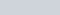And the work done by a variable force is defined as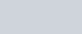Now we are having a system as shown in the figure. consisting of spring with one end connected to a wall and another end is connected to a block of mass say “m”. Spring is ideal.

Now we take the x and y-axis for study as the horizontal and vertical axis and we are in an inertial frame of reference. Now we displace the block of mass m which is connected to spring towards +ve x-axis a distance x i.e the elongation in spring from natural length is “x”.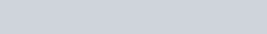as force acts opposite to displacement asangle between force and Displacement is 180 degrees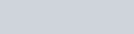Limits  of displacement “x” in  integration are from 0 to xSince we stretched the spring from natural length i.e. x= 0 to x= x .

Now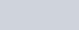The above expression gives the amount of work done by the ideal spring, whether compressed or elongated by a distance “x”.

Stay tuned with Laws Of Nature for more interesting and useful content.